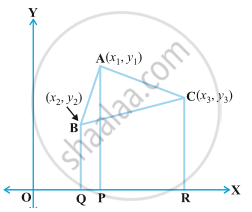Karnataka Secondary Education Examination BoardKarnataka SSLC
Share

# Area of a Triangle

#### description

We know that Area of a triangle = 1/2 xx "base" xx "altitude", but how to find area of a triangle on a plane? Here, we will learn the derivation of a formula to find area of triangle on plane.

#### notesGiven: O is the origin of X-axis and Y-axis.
A(x_1,y_1), B(x_2,y_2) and C(x_3,y_3) are the coordinates.
To find: area of ∆ ABC
Construction: Draw BQ, AP and CR ⊥ X-axis.
Proof: Since, BQ, AP and CR ⊥ X-axis
∠Q= ∠P= ∠R= 90°
we know that if corresponding are equal then the lines are parallel
thus BQ, AP and CR are parallel to each other.
Hence, ABQP, APRC and BQRC are trapeziums because as per the properity of trapezium, a pair of sides is parallel to each other and the other pair is non-prallel to each other.
area of trapezium = 1/2 (sum of parallel sides)(Shortest distance between them)
ar(∆ ABC)= area of trapezium ABQP+ area of trapezium APRC- area of trapezium BQRC

= 1/2 (BQ+AP)(QP)+ 1/2 (AP+RC)(PR)- 1/2 (BQ+RC)(QR)

= 1/2 [(y_2+y_1)("OP"-"OQ")+ (y_1+y_3)("OR"-"OP")- (y_2+y_3)("OR"-"OQ")]

= 1/2 [(y_2+y_1)(x_1-x_2)+ (y_1+y_3)(x_3-x_1)- (y_2+y_3)(x_3-x_2)]

= 1/2 [y_2x_1- y_2x_2+ y_1x_1- y_1x_2+ y_1x_3- y_1x_1+ y_3x_3- y_3x_1- y_2x_3+ y_2x_2- y_3x_3+ y_3x_2]

= 1/2 [y_2x_1- y_1x_2+ y_1x_3- y_3x_1- y_2x_3+ y_3x_2]

ar(∆ ABC)=1/2 [x_1(y_2-y_3) +x_2(y_3-y_1) +x_3(y_1-y_2)]

### Shaalaa.com

Coordinate Geometry part 11 (Area of triangle) [00:10:14]
S
0%